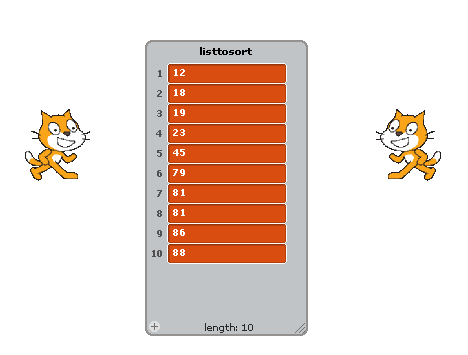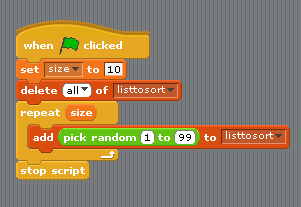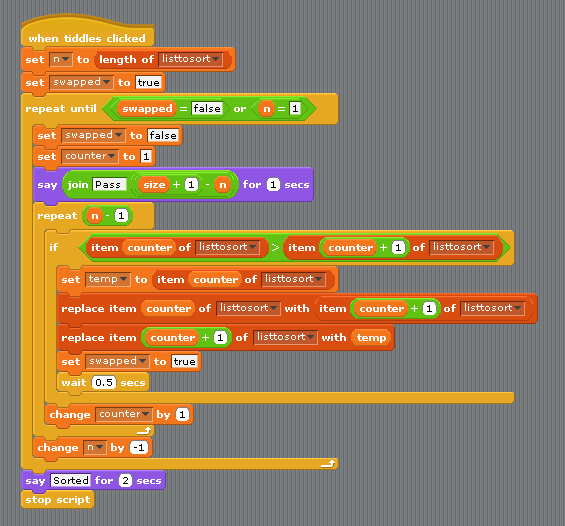# Scratch ProgrammingBubble Sort

## Introduction

Sorting data is a key task in computer programming. Over the years, many algorithms have been written that achieve this task, all with varying degrees of efficiency. The bubble sort is one of the techniques that is most straightforward to implement.

Here is a pseudocode algorithm for the Bubble Sort,

`Repeat    swapflag = false    For count = 1 To N - 1       If bubbleItem[count]>bubbleItem[count + 1] Then          temp = bubbleItem[count]          bubbleItem[count] = bubbleItem[count + 1]          bubbleItem[count + 1] = temp          swapflag = true       End If    Next    N = N - 1 Until swapflag = false Or N = 1`

## The CodeThis first section of code runs when the green flag is clicked and creates a list of 10 random numbers.This section of code performs the bubble sort with some pausing and output to show what is happening to the numbers in the list as they are sorted.The two cat sprites have similar scripts, the cat on the right just does the sorting without talking.

## Challenges

There are lots of different ways to sort data in a list. Here are some pseudocode descriptions of some of them,

### Insertion Sort

`For outerLoop = 1 To arrayLength - 1    temp = item[outerLoop]    innerLoop = outerLoop    While (innerLoop > 0 And item[innerLoop - 1] >= temp)       item[innerLoop] = item[innerLoop - 1]       innerLoop = innerLoop - 1    End While    item[innerLoop] = temp Next outerLoop`

### Selection Sort

`For outerLoop = 0 To arrayLength - 2    minValue = outerLoop    For innerLoop = outerLoop + 1 To arrayLength - 1       If item[innerLoop] < item[minValue] Then minValue = innerLoop    Next innerLoop    temp = item[outerLoop]    item[outerLoop] = item[minValue]    item[minValue] = temp Next outerLoop`

### Shell Sort

`h = 1 While (h<=arrayLength/3)    h = h * 3 +1 End While While (h > 0)    For outerLoop = h To arrayLength - 1       temp = item[outerLoop]       innerLoop = outerLoop       While (innerLoop > h - 1 And item[innerLoop - h] >= temp)          item[innerLoop] = item[innerLoop - h]          innerLoop = innerLoop - h       End While       item[innerLoop] = temp    Next outerLoop    h = (h - 1)/3 End While`

These are all quite tricky to implement. Have a go at whichever one takes your fancy and test that it actually sorts the data correctly. Then use a list with more numbers and compare the speed of each algorithm.